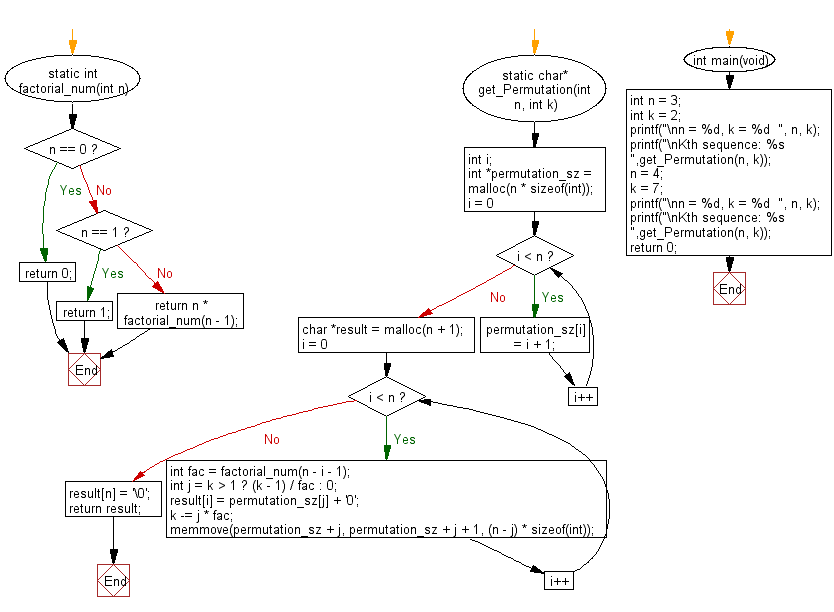﻿ C Program: kth permutation sequence from n and k

# C Exercises: Get the kth permutation sequence from two given integers n and k

## C Programming Mathematics: Exercise-5 with Solution

The following set contains a total of n! unique permutations
Set: [1, 2, 3, ..., n]
If n =3 we will get the following sequence:
1. "123"
2. "132"
3. "213"
4. "231"
5. "312"
6. "321"
Input: n = 3, k = 4
Output: "231"
Write a C program to get the kth permutation sequence from two given integers n and k. In these integers, n is between 1 and 9 inclusive. In addition, k is between 1 and n! Inclusive.

Example:
Input:
n = 3
int k = 2

n = 4
k = 7
Output:
Kth sequence: 132
Kth sequence: 2134

Sample Solution:

C Code:

``````#include <stdio.h>
#include <stdlib.h>
#include <string.h>

static int factorial_num(int n)
{
if (n == 0) {
return 0;
} else if (n == 1) {
return 1;
} else {
return n * factorial_num(n - 1);
}
}

static char* get_Permutation(int n, int k)
{
int i;
int *permutation_sz = malloc(n * sizeof(int));
for (i = 0; i < n; i++) {
permutation_sz[i] = i + 1;
}

char *result = malloc(n + 1);
for (i = 0; i < n; i++) {
int fac = factorial_num(n - i - 1);
int j = k > 1 ? (k - 1) / fac : 0;
result[i] = permutation_sz[j] + '0';
k -= j * fac;
memmove(permutation_sz + j, permutation_sz + j + 1, (n - j) * sizeof(int));
}
result[n] = '\0';
return result;
}

int main(void)
{
int n = 3;
int k = 2;
printf("\nn = %d, k = %d  ", n, k);
printf("\nKth sequence: %s ",get_Permutation(n, k));
n = 4;
k = 7;
printf("\nn = %d, k = %d  ", n, k);
printf("\nKth sequence: %s ",get_Permutation(n, k));
return 0;
}
```
```

Sample Output:

```n = 3, k = 2
Kth sequence: 132
n = 4, k = 7
Kth sequence: 2134
```

Flowchart:C Programming Code Editor:

Improve this sample solution and post your code through Disqus.

What is the difficulty level of this exercise?

Test your Programming skills with w3resource's quiz.

﻿